# Determination of price and quantity supplied by monopolistic firm in the short run

This article describes the process by which a monopoly firm, i.e., a firm that is the only firm selling a particular commodity, selects the quantity to produce and the price to set for the commodity.

Here are some key features of a monopoly firm:

1. The firm is a price setter rather than a price taker -- it can choose any price it wants.
2. However, for a given price set by the firm, and a given quantity that the firm produces, there is no guarantee that the firm will be able to sell all of what it produces. Thus, instead of being told a market price and trying to optimize the quantity, the firm needs to optimize a (price,quantity) pair based on the market demand curve.

The situation is in sharp contrast to that of determination of quantity supplied by firm in perfectly competitive market.

The situation is similar to determination of quantity supplied by firm in oligopoly. In the latter, however, the firm also has to be concerned about the behavior of competing firms, and the response these firms may show to price changes by the firm. Oligopolistic competition is typically studied using tools of game theory.

More generally, when a firm has market power, it has some leeway in setting prices but, in return, has to explicitly consider the market demand curve it faces. When a firm has monopoly, the leeway in setting prices as well as the importance of the market demand curve are maximal.

## Similar determinations

### Contrast with firm in perfectly competitive market

There are three key differences. The first two are related and the third follows from them:

Parameter Firm in perfectly competitive market Monopolistic firm
control over market price no control. The firm must accept the market price. significant control. The firm sets both its price and quantity of production.
guarantee of selling everything it produces guaranteed, as long as it prices at the market price. no guarantee. The firm needs to choose its price appropriately based on its estimate of the nature of demand and the quantity it produces.
nature of marginal revenue curve (marginal revenue plotted on the vertical axis as a function of quantity produced on the horizontal axis) flat horizontal curve with price coordinate equal to the market price it's complicated, but generally, a downward sloping curve. Roughly speaking, it represents the demand curve facing the firm.

Another way of putting these is that monopolistic firms have more power (in the sense of setting prices) but also more responsibility (in the sense of having to figure what price to set).

Most of the analysis here can be transferred to the perfectly competitive case, with the following change: the marginal revenue curve is replaced by a horizontal curve at value equal to the market price.

## Law of one price assumption and price discrimination

In the discussion here, we assume the law of one price, i.e., that the firm must offer a single price to all those buying from it. In other words, we assume that it is impossible for the firm to practice price discrimination, i.e., charge different prices to different buyers.

If the firm can practice price discrimination, the analysis changes considerably. The key significance is that with price discrimination, the firm can charge higher prices for buyers with higher reservation prices and thus avoid to some extent the trade-off between expanding its market and lowering its price.

## Cost and revenue definitions

We assume that the firm has two costs:

• Fixed costs are fixed in advance and cannot be altered in the short run. These may include investments in land or other fixed assets that cannot be acquired or got rid of quickly.
• Variable costs are costs that vary with the quantity produced.

We define:

Cost or revenue notion Acronym Definition How does it vary with quantity?
Fixed cost FC Cost of expenditure on fixed factors of production that cannot be varied in the short run Independent of quantity
Variable cost VC Cost of expenditure on factors of production that can be varied in the short run Increases with quantity
Total cost TC Fixed cost + Variable cost Increases with quantity
Average cost ATC, AC (Total cost)/Quantity Generally, first decreases and then increases with quantity
Average fixed cost AFC (Fixed cost)/Quantity Decreases with quantity (by definition, because denominator is increasing and numerator is constant).
Average variable cost AVC (Variable cost)/Quantity Generally, increases with quantity when quantity is large enough. For small quantities, it may decrease with quantity.
Marginal cost MC Derivative of total cost with respect to quantity; since fixed cost is independent of quantity, this is the same as the derivative of variable cost with respect to quantity Generally, increases with quantity when quantity is large enough. For small quantities, it may decrease with quantity.
Revenue R Revenue earned by producing a given quantity of the commodity. It equals the product of quantity produced and the (unit) market price. Note that because of the small size of the firm relative to the market, it must sell everything it produces at the market price Increases with quantity (by definition).
Price P Price chosen by the firm See discussion below
Marginal revenue MR Depends on quantity
Profit (not accounting for fixed costs) Equals R - VC, same as Q(P - AVC) where Q is the quantity produced, P is the unit price chosen by the firm, and AVC is the average variable cost at the given value of Q.
Profit (accounting for fixed costs) Equals R - TC, same as Q(P - ATC), where Q is the quantity produced, P is the unit price, and AC is the average (total of fixed and variable) cost. In the short run, the firm may choose to produce even if the profit, after accounting for fixed costs, is negative.

## Calculation problem for the firm

### Information available to the firm

As before, we assume that the firm serves an entire market and has no competing firms. The firm has two pieces of information:

Piece of information Nature Equivalent pieces of information How it's decided
the marginal cost curve of the firm This is a curve describing the marginal cost as a function of quantity produced. Traditionally, the quantity is plotted along the horizontal axis and the cost function along the vertical axis. It is not a single number but rather a functional relationship. Knowledge of the marginal cost curve is mathematically equivalent to knowledge of the average variable cost curve, the variable cost curve, and (subject to knowledge of the fixed cost),the average fixed cost curve, the average total cost curve, and the total cost curve. This means that knowledge of any one of these curves can be used to deduce the others. Determined by the firm's production technology and factors of production. The curve itself is not subject to change.
the market demand curve facing the firm This is the market demand curve that plots the quantity demanded by the market the firm serves as a function of the price. See determinants of demand for details.

### Goal of the firm

The firm has to choose two things:

• The quantity that it produces. Call this$Q$.
• The price at which it sells whatever it produces. Call this$P$.

The goal of the firm is to maximize its profit, which (not accounting for fixed costs) is given by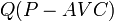$Q(P - AVC)$ where$Q$ is the quantity produced,$P$ is the price chosen by the firm, and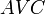$AVC$ is the average variable cost (dependent on$Q$.

### Note on conventions for profit: accounting and not accounting for fixed costs

If$Q$ denotes the quantity produced,$P$ denotes the market price (which is also the same as the marginal and average revenue) then the total revenue is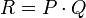$R = P \cdot Q$, and the total variable cost is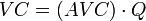$VC = (AVC) \cdot Q$ where$AVC$ is the average variable cost for the given choice of$Q$. The net profit (not accounting for fixed costs) is$R - VC$, which becomes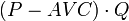$(P - AVC) \cdot Q$.

In particular, the net profit (not accounting for fixed costs) is zero at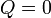$Q = 0$.

The net profit accounting for fixed costs is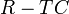$R - TC$, which becomes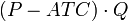$(P - ATC) \cdot Q$, where$AC$ is the average total cost, including both average fixed costs and average variable costs.

This is smaller than the profit calculated by not accounting for fixed costs. Also, for the short-run choices, this profit value may well be negative, because the firm treats its fixed costs as sunk costs.

Note that as far as the maximization problem is concerned, both choices of the profit function are equivalent, because they differ by a value that is constant (in the short run). However, there is an advantage to choosing the convention where we compute profits not accounting for fixed costs. The advantage is that it computes the profits relative to the situation of staying idle, i.e., producing nothing, and hence, can be more useful in quickly figuring out whether a certain quantity of production is more profitable than producing nothing.